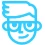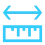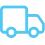Question

# Tiered Vendor discounts

• 9 replies
• 127 views

Userlevel 3• Freshman I
• 28 replies

Is there a way in current functionality to set up tiered vendor discounts?

I have this case

If PO total amount is from 0 to 10,000 then apply a 15% discount

if PO total amount is  100,000 then apply the 15% discount to the first 10,000 and apply 10% to the remaining 90,000

if PO total amount is  500,000 then apply the 15% discount to the first 10,000, then apply 10% to 90,000 and then apply 5% to the remaining 400,000 and so on

Thanks

### 9 replies

Userlevel 7+15

Hi @nperez94naw

Here is how Acumatica handles vendor tier discounts:

# Calculation of Document-Level Discounts with Tiers Defined by Amounts

For document-level vendor discounts, which are unconditional, the discount tiers can be created only by break points defined as amounts, and the discount can be defined as a percentage of the document amount or as a fixed amount. When the system processes a purchase order or a bill and finds a qualifying discount sequence the discountable amount is compared to the break points in the discount sequence as follows:

1. The document amount is compared to the first break point. If the document amount is less than the amount of the first break point in the sequence, the discount equals zero, and further comparison is not performed.
2. The document amount is compared to a next break point. If the document amount is greater than or equal to the amount of any break point but less than the amount of the next break point (or greater than the amount of the last break point), the system calculates the discount as follows:
• If the discount is a fixed amount, the fixed amount specified as the discount for this tier is deducted from the document amount.
• If the discount is a percentage, the percent (specified for this tier) of the document amount is calculated and deducted from the document amount.
• Further comparison is not performed.
3. Comparison continues similarly, with Step 2 repeated until a discount is found or the last break point is checked.

The examples below illustrate the way these document-level discounts are calculated.

Example 1: If a sequence for a document-level discount has tiers defined by amounts and the discounts are expressed as percentages, the discount amount corresponding to the appropriate tier is subtracted from the document total. Suppose that the sequence is specified with the following break points:

• \$1000: 5%
• \$2000: 7%
• \$5000: 10%

For a document with a \$900 total, the discount is \$0. For a document with a \$2500 total, the discount is \$175 (7%), and for a document with a total of \$9000, the discount is \$900 (10%).

Example 2: Suppose that a sequence for a document-level discount has tiers defined by amounts, with the discounts expressed as fixed amounts that are subtracted from the total amount of the document. Further suppose that the sequence is specified with the following break points:

• \$1000: \$100
• \$2000: \$225
• \$3000: \$350

For purchase orders with amounts less than \$1000, your company receives a discount of \$0. The discount for an order with a total between \$1000 and \$1999.99 is \$100, the discount on an order between \$2000 and \$2999.99 is \$225, and the discount for an order of \$3000 or more is \$350.

Userlevel 3Hello @kbeatty21

thank you so much for your feedback.

I am trying to set it up the way that says in the example No. 2 but I can’t figure out how.

Where do set it up as tiered discounts?

The way I have it ( in the screenshot below), if the PO total is 2500 the discount amount is 175 which is 2500*.07

Userlevel 7+15

Hi @nperez94naw

How is your discount set up? It appears since you have Item Price Classes that yours is set up by line. To do what you are looking for you need to set up based on document. See the screenshots below:

Userlevel 7+15

HI @nperez94naw

I realized just now you asked about a PO and I posted a Bill but to show you, it works the same on PO’s as well.

Userlevel 3Hi @kbeatty21

I realized that I am doing it right, but those are not the results I need.

In the example you are showing, AP bill for 115,000, the discounts I am looking for the system to calculate are as follow.

The first 5,000 →  2% = 100.00

the other 5,000 (from 5,000.01 to 10,000)  → 3% = 150.00

The other 90,000 (10,000.01 to 100,000.00) → 4% = 3,600.00

The remaining 15,000 (100,000.01 - 115,000.00) → 0% = 0.00

So the total discount will be (100+150+3600+0) = 3,850

Userlevel 7Hi @nperez94naw - were you able to find a solution? Thank you!

Userlevel 3Hi @Chris Hackett

No I was not able and I don’t think there is a way.

Userlevel 6+1

Hi @nperez94naw,

I suggest that you post this as a New Idea.

The tiered discounts as answered by @kbeatty21 looks at the total amount of the document and applies the percentage defined for that tier.

-Dana

Userlevel 3Thank you @Dana Moffat

I did it now. ;)